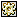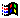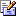Contents Articles Behaviors Books Director News Director Web Sites FAQ Games Mailing Lists News Groups Project Examples Reviews Software Tools Useful Web Sites Utilities Xtras

 Don't miss these#Draw a line with lingo

 Compatibilities:Rating:Author: ColeTierney

Here is an example of a lingo draw line routine From Cole Tierney"s Code Corner http://homepages.together.net/%7Etierney/cole/code/

 -- This example expects sprite 1 to be a line shape, -- castmember 1 to be a line shape going from upper left to bottom right, -- and castmember 2 to be a line shape going from bottom left to top right. -- -- Sample usage:  drawLine point( 10, 5 ), point( 100, 230 ) on drawLine p1, p2     -- First, sort the points.     if the locH of p1 < the locH of p2 then         set x1 = the locH of p1     set y1 = the locV of p1         set x2 = the locH of p2     set y2 = the locV of p2       else         set x1 = the locH of p2     set y1 = the locV of p2         set x2 = the locH of p1     set y2 = the locV of p1       end if     -- Check for boundry conditions.   -- (Vertical and Horizontal lines need to be atleast one pixel wide.)     if x1 = x2 then set x2 = x2 + 1   if y1 = y2 then set y2 = y2 + 1       if y1 < y2 then         set the memberNum of sprite 1 = 1     set the rect of sprite 1 = rect( x1, y1, x2, y2 )     else         set the memberNum of sprite 1 = 2     set the rect of sprite 1 = rect( x1, y2, x2, y1 )       end if     updatestage   end

 Contact MMI 36 South Court Sq Suite 300 Newnan, GA 30263 USASend e-mail